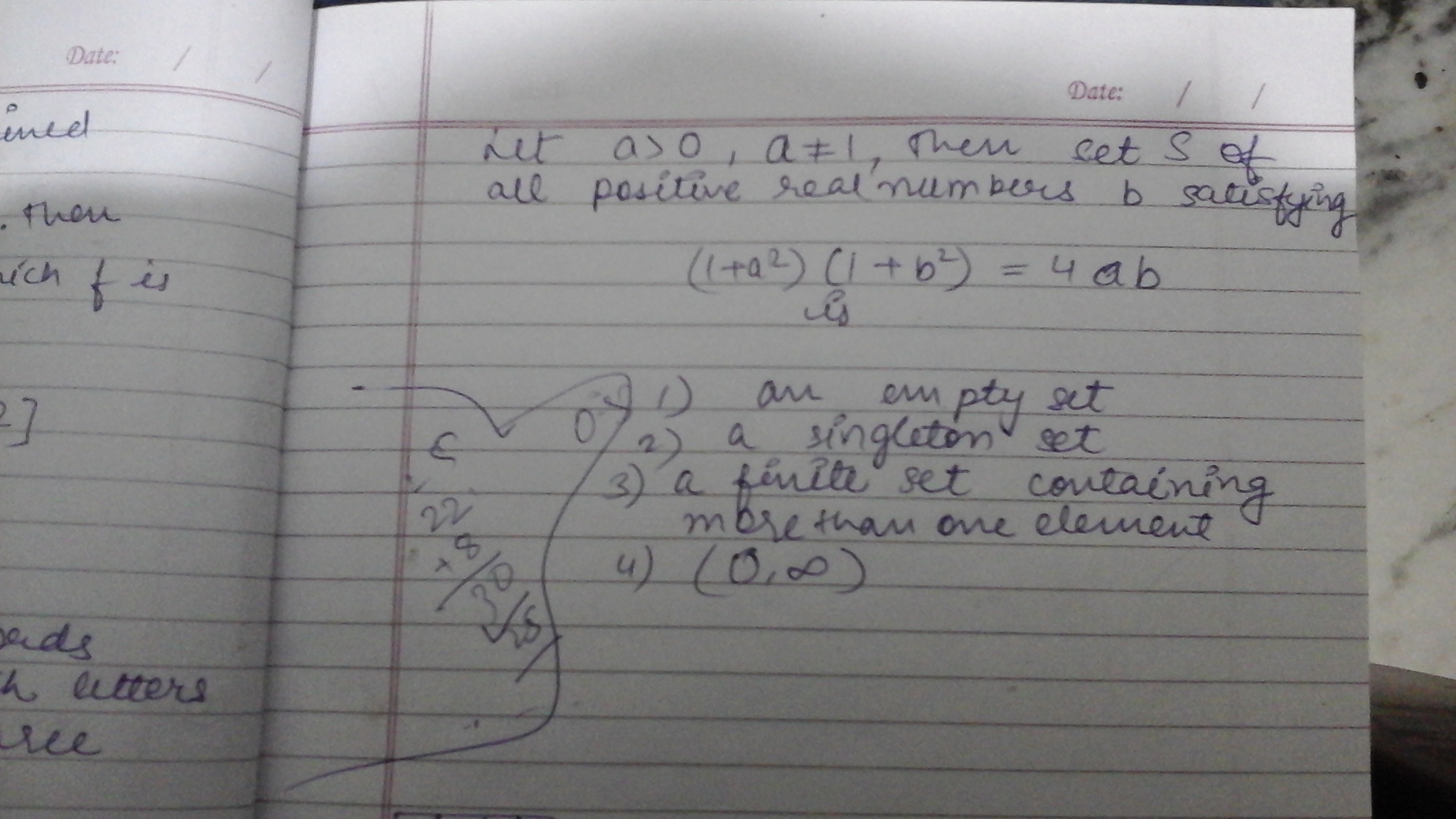# Doubt from setsGiven a>0 and a\neq 1 and (1+a^2)(1+b^2)=4ab

That means from \displaystyle b=\frac{(1+a^2)(1+b^2)}{4a}>0

So using Arithmetic Geometric Inequality

we have 1+a^2\geq 2a. and 1+b^2\geq 2b.

\Longrightarrow (1+a^2)(1+b^2)\geq 4ab, Equality hold a=b=1

But above given that a\neq 1\Rightarrow b\neq 1.

So no positive no. b for which (1+a^2)(1+b^2)=4ab.

1 Like